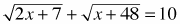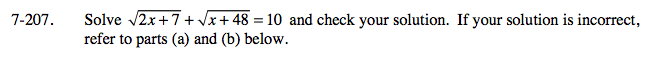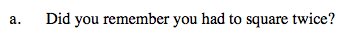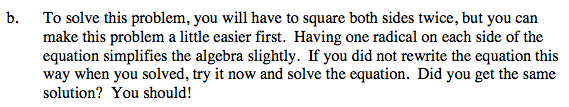Home > A2C > Chapter 7 > Lesson 7.3.4 > Problem7-207

7-207.
1. Solveand check your solution. If your solution is incorrect, refer to parts (a) and (b) below. Homework Help ✎

1. Did you remember you had to square twice?

2. To solve this problem, you will have to square both sides twice, but you can make this problem a little easier first. Having one radical on each side of the equation simplifies the algebra slightly. If you did not rewrite the equation this way when you solved, try it now and solve the equation. Did you get the same solution? You should!x = 1 (x = 681 gives an impossible answer when checked)